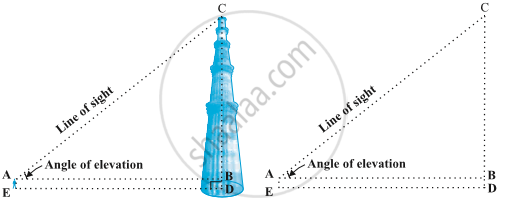# Heights and Distances

#### description

• Problems involving Angle of Elevation
• Problems involving Angle of Depression
• Problems involving Angle of Elevation and Depression

#### notes

In everyday life we come across many buildings, monuments and other structures. The heights of these structures can be found out with the help of trigonometry.In this figure, the line AC drawn from the eye of the student to the top of the minar is called the line of sight. The student is looking at the top of the minar. The angle BAC, so formed by the line of sight with the horizontal, is called the angle of elevation of the top of the minar from the eye of the student.

Thus, the line of sight is the line drawn from the eye of an observer to the point in the object viewed by the observer. The angle of elevation of the point viewed is the angle formed by the line of sight with the horizontal when the point being viewed is above the horizontal level, i.e., the case when we raise our head to look at the object.

You would need to know the following:

(i) the distance DE at which the student is standing from the foot of the minar

(ii) the angle of elevation, BAC, of the top of the minar

(iii) the height AE of the student.

Assuming that the above three conditions are known, how can we determine the height of the minar?

In the figure, CD = CB + BD. Here, BD = AE, which is the height of the student.

To find BC, we will use trigonometric ratios of BAC or A. Our search narrows down to using either tan A or cot A, as these ratios involve AB and BC.

Therefore, tanA= "BC"/"AB" or cotA= "AB"/"BC", which on solving would give us BC. By adding AE to BC, you will get the height of the minar.

Now, consider the situation given below, The girl sitting on the balcony is looking down at a flower pot placed on a stair of the temple. In this case, the line of sight is below the horizontal level. The angle so formed by the line of sight with the horizontal is called the angle of depression.

Thus, the angle of depression of a point on the object being viewed is the angle formed by the line of sight with the horizontal when the point is below the horizontal level, i.e., the case when we lower our head to look at the point being viewed

Example 1 : A tower stands vertically on the ground. From a point on the ground, which is 15 m away from the foot of the tower, the angle of elevation of the top of the tower is found to be 60°. Find the height of the tower.

Solution : First let us draw a simple diagram to represent the problem (see Figure). Here AB represents the tower, CB is the distance of the point from the tower and ∠ ACB is the angle of elevation. We need to determine the height of the tower, i.e., AB. Also, ACB is a triangle, right-angled at B.

To solve the problem, we choose the trigonometric ratio tan 60° (or cot 60°), as the ratio involves AB and BC.

Now, tan 60° = "AB"/"BC"

sqrt3= "AB"/ 15

"AB"= 15 sqrt3

Hence, the height of the tower is15 sqrt3 m.

If you would like to contribute notes or other learning material, please submit them using the button below.

### Shaalaa.com

Application of Trigonometry part 1 (Concepts) [00:13:33]
S